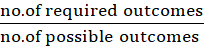### Probability

Mathematics J.S.S 2 Third Term

Theme: Mensuration and Geometry

Sub Theme: Shapes

WEEK 10

Probability

Performance Objectives

Students should be able to;

1. Discuss the occurrence of chance events in everyday life
2. Determine the probability of certain events
3. Apply occurrence of chance events/probabilities in everyday life

Probability

Content

Probability is a measure of the likelihood of an event happening, that is, the likelihood of a required outcome. The required outcomes are the required possibilities in an occurrence or happening.

In the form of a fraction, Probability = no. of required outcomesno. of possible outcomesView More